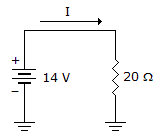# Electronics - Ohm's Law

### Exercise :: Ohm's Law - General Questions

16.

A battery's capacity to deliver power is measured in

 A. kWh B. Ah C. Wh D. Vh

Answer: Option B

Explanation:

No answer description available for this question. Let us discuss.

17.

Power supplies are regulated to prevent the output voltage from changing due to changes in the

 A. line voltage B. supply load C. frequency D. both line voltage and supply load

Answer: Option D

Explanation:

No answer description available for this question. Let us discuss.

18.What is the power in the given circuit?

 A. 3.92 kW B. 280 W C. 28.6 W D. 9.8 W

Answer: Option D

Explanation:

No answer description available for this question. Let us discuss.

19.

If current through a resistance is halved

 A. the resistance is halved B. the voltage is halved C. the voltage doubles D. none of the above

Answer: Option B

Explanation:

No answer description available for this question. Let us discuss.

20.

Ohm's law is a relationship between

 A. voltage, current, and time B. power, current, and resistance C. resistance, time, and current D. voltage, current, and resistance

Answer: Option D

Explanation:

No answer description available for this question. Let us discuss.

#### Current Affairs 2021

Interview Questions and Answers